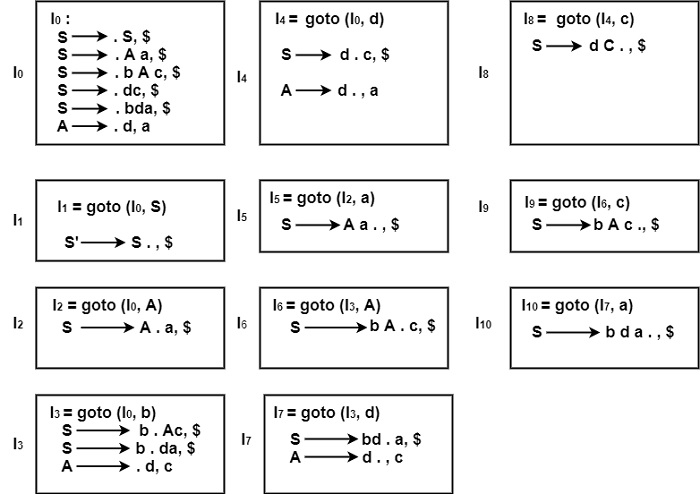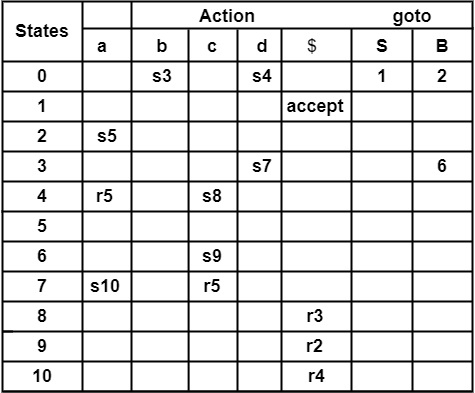# Constructing LALR (1) Parsing table for a given grammar. Problem&minus; Construct LALR (1) Parsing table for the grammar. S → A a|b A c|dc|bda A → d Parse input string "bdc" using LALR Parsing Table.

Compiler DesignProgramming LanguagesComputer Programming

## Solution

The first number the production as below −

Step1− Construct Augmented Grammar

(0) S′ → S

(1) S → A a

(2) S → b A c

(3) S → d c

(4) S → b d a

(5) A → d

Step2− Find Closure & goto. Find the canonical set of LR (1) items for the Grammar.In the states, I0 to I10, no states have a similar first element or core. So, we cannot merge the states. Some states will be taken for building the LALR parsing table.

LALR Parsing TableParsing of String "bdc"

StackInput StringAction
$0bdc$Shift 3
$0 b 3dc$Shift 7
$0 b 3 d 7c$Reduce by A → d
$0 b 3 A 6c$Shift 9
$0 b 3 A 6 c 9$Reduce by S → b Ac
$0 s 1$accept

Construction of LALR Parsing Table

Algorithm

Input− Augmented Grammar G′

Output− LALR Parsing Table

Method

• Construct LR (1) set of items, i.e., construct

C = {I0, I1, I2 … . . In}

• Select the similar states having the same core, or first component and merge them into one.

Let C′ = {J0, J1, J2 … . . Jm} be the resulting set.

• Construct Parsing Action for state J1 similar to CLR construction. If there is a conflict in the Parsing Table, the algorithm can be considered to fail to produce an LALR parser.
• Construct goto actions as below.

Let goto [J,∗] = K where J is the union of one or more states of C.

i.e., J = I1 ∪ I2 … .∪ Im, then

then K = goto (I1,∗) ∪ goto (I2,∗) … .∪ goto (Im,∗)